### Home > PC > Chapter 1 > Lesson 1.1.1 > Problem1-9

1-9.
1. Write each product as a power of 2. Then check your results using your chart. Homework Help ✎

 Example: 23 · 2−2 = Solution: 23 · 2−2 = 21 Check: 8 ·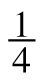= 2
1. 2−4 · 22 =

2. 2−1 · 2−2 =

3. 20 · 2−3 =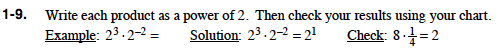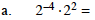Solution:
2−4 · 22 = 2−2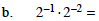Follow the steps in the example.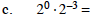Follow the steps in the example.